# A useful method for model-building II: Synthesizing response functions from individual components

williamghunter.net > Articles > A useful method for model-building II

by William G. Hunter and Andrzej P. Jaworski

February 1986

Practical Significance

There is a vast difference between quality control and quality improvement, passive statistical tools such as Shewhart control charts are useful for quality control, to determine whether the process under surveillance shows any signs of going out of its state of statistical control. On the other hand, more active tools are needed for quality and productivity improvement. In order to improve a process or a product, it is often helpful to use experimental designs in developing a mathematical equation or set of equations to relate the response(s) of interest to important process and environmental variables. Such models can aid in understanding how the relevant processes work so they can be modified in desirable ways. This report contains a practical suggestion that model-builders may find helpful. It involves synthesizing response functions of interest by starting with the simpler task of constructing models for component responses or subsets of them.

Key Words: Model-building, response surfaces, factorial design, component responses, synthesizing models.

Abstract

In model-building investigations the response of interest y is often a function of two or more components. The usual procedure in such situations is to use this function to compute the values of y for each run and then obtain the fitted equation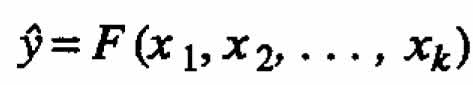?relating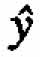to relevant process variables. In this paper we suggest a different method that will sometimes be preferable: first fit equations to the component responses (either individually or in subsets) and then combine this these equations to obtain the desired function F. The proposed method is especially useful when the usual procedure fails to produce a satisfactory model. A chemical example illustrates how, by using this method, a second-order model can be obtained from a first-order design (a two-level factorial design).

1. Introduction

Frequently in model-building work the most important dependent variable is not measured directly but is calculated from other quantities. For example, (1) yield is sometimes computed by multiplying the recorded values of conversion and selectivity, and (2) profit is usually calculated from several measured quantities.

Using a simple chemical example for illustration, we show that useful models can sometimes be developed by fitting equations to more primitive measurements and then manipulating these equations to construct a model for the dependent variable (response) of interest. This method is in contrast to the standard procedure of first calculating the value of the response of interest for each experimental run and then fitting an equation to these values. By employing the method we propose, an investigator may be able to construct more flexible models that can provide better estimates of the responses and greater insight into the behavior of the system under study.

A model is a set of one or more equations that represents the behavior of a particular physical or biological system. Model-building is the process, typically iterative, by which investigators attempt to construct an adequate model. An essential part of this process is checking a candidate model against data to determine whether there is serious lack of fit Statistical procedures are most useful when they aid not only in detecting such lack of fit but also in pinpointing its possible source, so that research workers are helped in the next stage of creating a better model (Box and Hunter, 1962). Models can be discussed in terms of being either primarily empirical or mechanistic (see, for example, Box, Hunter, and Hunter (1978)), or a mixture of the two. This last class can be called empirical-mechanistic (see, for example, Phadke, Box, and Tiao (1977)).

After studying a specific empirical model-building problem in Section 2 to illustrate the basic idea of the present paper, we discuss the more general situation in Page 5 Section 3. In Section 4 we address some problems of inference, especially as it relates to computation of the variance of the estimated response. Concluding remarks are given in Section 5, and mathematical details are relegated to appendices.

2. Chemical example

Consider the simple chemical reaction equation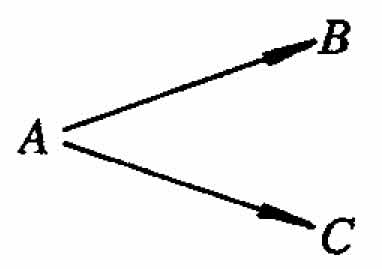where A is the reactant, B is the product, and C is the by-product. Suppose a chemist is studying the effect of two controllable variables, temperature and time, on the yield of this reaction. Let x1 and x2 denote the coded values of these controllable variables. Suppose that a 22 factorial design with center point has been performed in random order, and the data shown in the first three columns of Table 1 are available.

Using regression analysis to fit a standard first-order response surface model, one gets: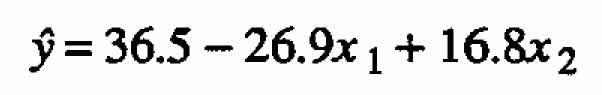(2.1)

Suppose it is known that the standard deviation of y is less than 1%. The contour lines for this first-order equation for selected values ofare shown in Figure 1(a) with the data points superimposed. It is evident from this figure as well as from the magnitude of the residual sum of squares (see column four in Table 1) that the first-order approximation is inadequate. If the model is assumed to include the interaction term, one obtains the following fitted equation:(2.2)

Contour lines for this model are shown in Figure 1(b). As would be expected, this response surface fits the data better than the first-order model however, there is still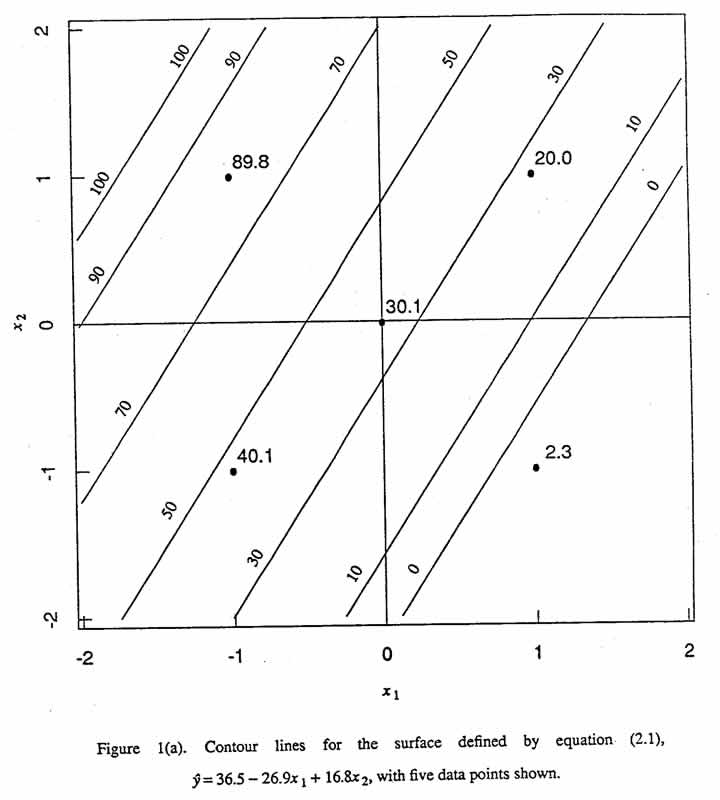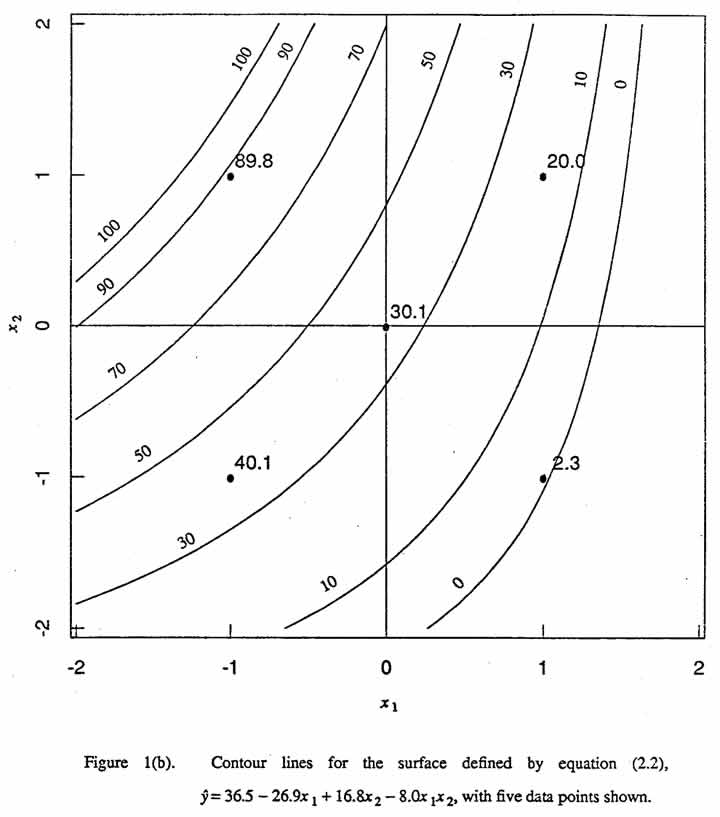significant lack of fit caused mainly by the curvature of the response surface (see column five in Table 1)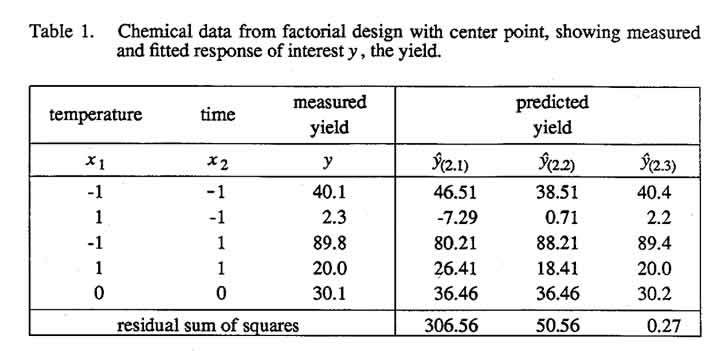Suppose that the raw data for this model-building problem were those shown in Table 2 and that the yield y for each run was calculated as the product of the corresponding measured values of conversion y1 and selectivity y2, that is, y = y1y2 (To define these terms,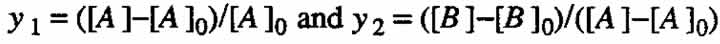, where the square brackets denote the concentration of a chemical constituent and the subscript zero denotes the initial value. Conversion is, then, the fraction of the initial amount of reactant A that has reacted, and selectivity is the fraction of the amount of reactant A that has reacted that has been converted into product B.) The values of y1, y2 and y lie between zero and one, and they are often given as percentages. Fitting first-order response surface models to y1, and y2 separately gives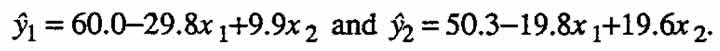Multiplying these two equations together and dividing by hundred to keep the yield results expressed in terms of percentages, we get: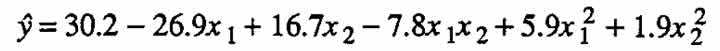(2.3)

Contour lines for this model are shown in Figure 1(c). There is no detectable lack of fit for this second-order model. A canonical analysis for this equation is given in Appendix A (see, in particular, Equation (A.4)).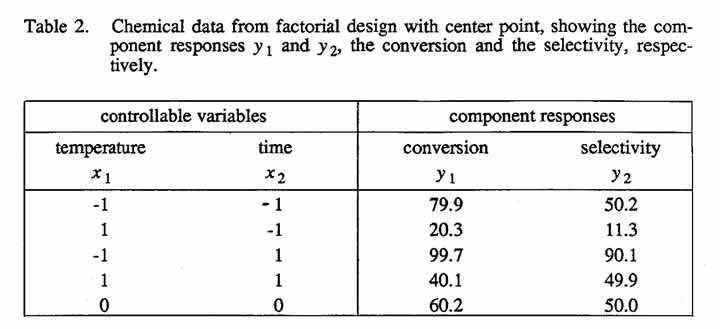(2.3)

Note that, by employing the above procedure, one is able to f?t a second-order model to data from a first-order design! Had the center point not been available, one could still have obtained a fitted second-order model.

We now examine some interesting aspects of special second-order models that are derived in this way. Suppose that there are two first-order equations,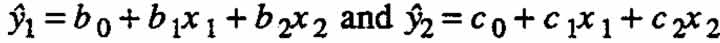and the response of interest is the product of these two component responses. Then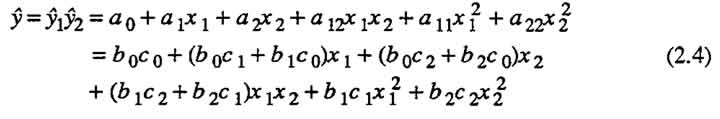Can a model derived in the manner described above characterize all possible second order response surfaces? The answer is no. For the case when there are two variables, the eigenvalues ←mbda;1 and ←mbda;2 associated with the matrix of second-order coefficients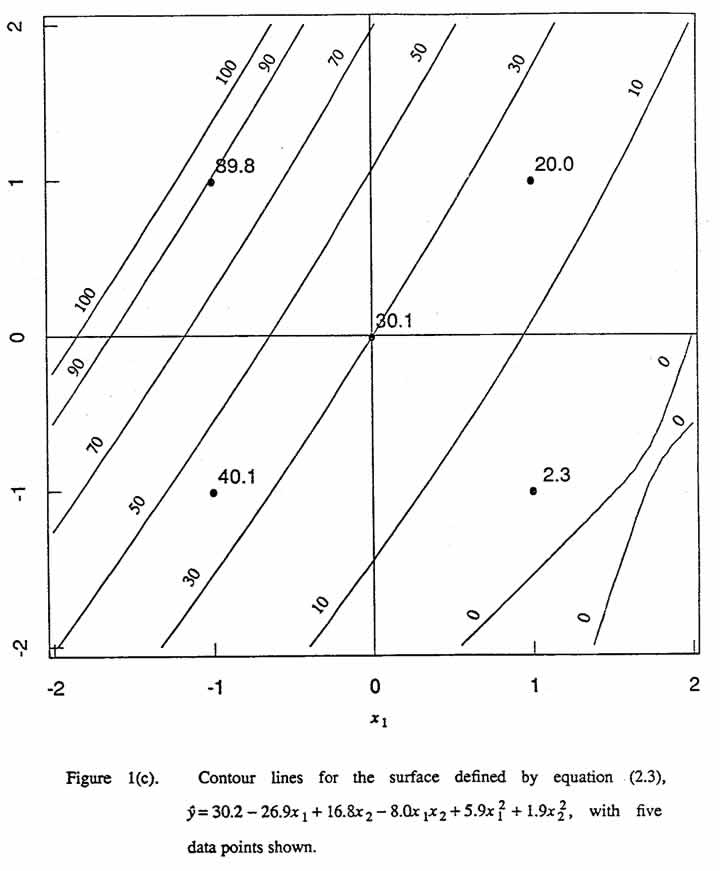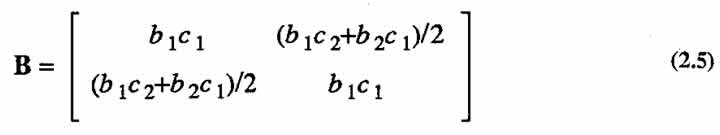are constrained to be of different signs, or else one or both of these values must be zero. In other words, a second-order model constructed as described above does not have complete flexibility in that the two eigenvalues cannot be both strictly positive or strictly negative. (For proof, see Appendix B.) This means that the response surfaces exhibiting minimum or maximum cannot be represented by the synthesized model. (For details of canonical analysis see, for example, Myers (1971).)

It is worthwhile to view this situation in the (←mbda;1, ←mbda;2) plane. Since the special second-order model (2.4) has eigenvalues of opposite sign or else at least one of the eigenvalues is zero, points cannot occur in the first or third quadrant of the (←mbda;1, ←mbda;2) plane. Four points are designated in Figure 2. Point (a), for (←mbda;1, ←mbda;2) = (0,0), corresponds to equation (2.1). Eigenvalues that are consistent with the general model form for a first-order equation with interaction fall along the dashed line. Point (b), for (←mbda;1, ←mbda;2) = (-4,4), corresponds to equation (2.2), an example of such a model. Point (c), for (←mbda;1, ←mbda;2) = (8.28, -0.48), corresponds to (2.3). The points (a), (b), and (c) in Figure 2, therefore, correspond to Figures 1(a), 1(b), 1(c), respectively. For more details on canonical analysis, see appendix A. To demonstrate flexibility of these special second order models, we show an additional contour map in Figure 1(d), which corresponds to point (d) in Figure 2.

Let us examine each one of these four cases. Here we use the standard notation (see, for example, Myers (1971)) in which a general second-order model is written as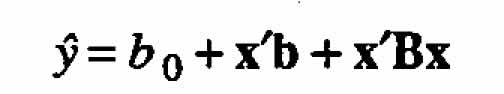For the case of only two independent variables the above is usually written as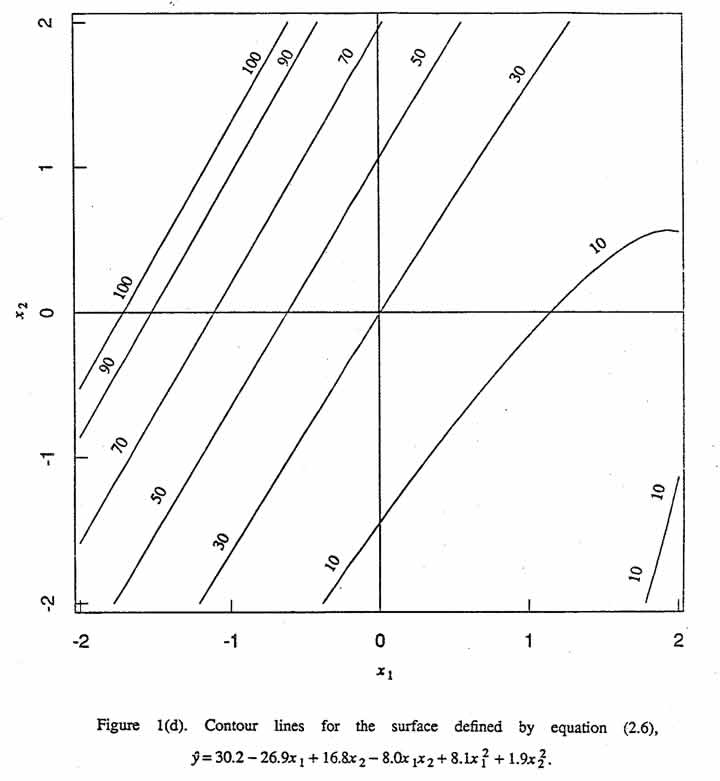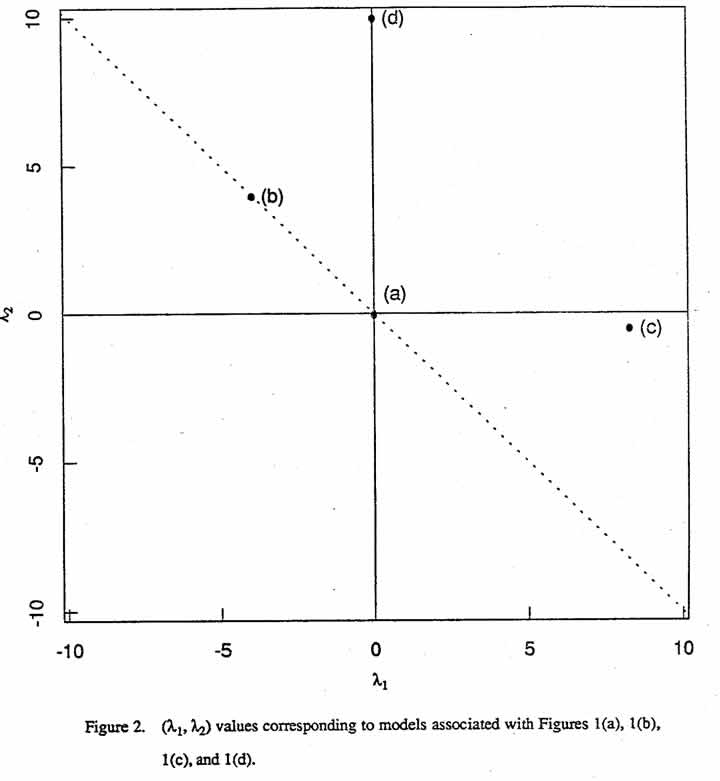Case (a) ←mbda;1 = ←mbda;2 = 0.

This condition implies that B = 0 and that the prediction equation is first-order. Figure 1(a) shows the contour lines of a surface for such an equation, namely (2.1). Note that, for equal increments in, these parallel straight lines must be equidistant from one another.

Case (b) -←mbda;1 = ←mbda;2 = 4.

In general, ←mbda;1 = -←mbda;2 implies that >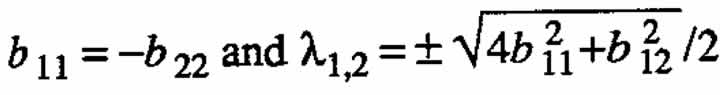. For the special case b11 = b22 = 0, one obtains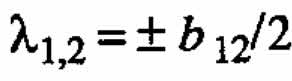. Figure 1(b) shows contour Unes of a surface for this special case, corresponding to equation (2.2), for which -←mbda;1 = ←mbda;2 = 4.

Case (c) -←mbda;1 = 8.28, ←mbda;2 = -0.48.